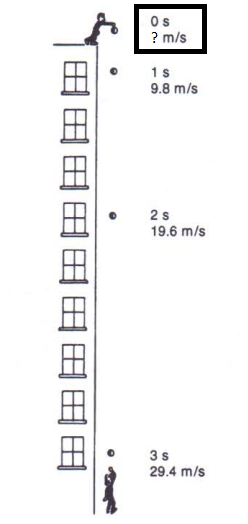# Gravity acceleration

Objects accelerate if drop freely near the surface of the earth
👁 247
statistics
calculateQuestions #: 7
Time:
Pass Score: 80.0%
Style
Mode

POINTS (1)

POINTS (1)

POINTS (1)

#### What is the speed of an object thrown upward with a starting speed of 29.4 at third second?POINTS (1)

#### What is the right equation relating acceleration, the distance travelled, and the time of fall is?

Given v0 is the original speed, t is the time elapsed, and a is gravity acceleration

1. d = v0t + ½ at2
2. d = v1t + ⅓ at * 2
3. d = v1t + ½ at * 2
4. d = v1t + ½ at * 3
POINTS (1)

#### What is the distance covered by an object falling from rest towards ground after 3 seconds?

Hint: use acceleration equation

d = v0t + ½ at2

POINTS (1)

POINTS (1)